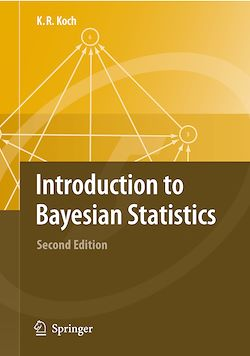# Introduction to Bayesian Statistics

-English

Description

This book presents Bayes’ theorem, the estimation of unknown parameters, the determination of confidence regions and the derivation of tests of hypotheses for the unknown parameters. It does so in a simple manner that is easy to comprehend. The book compares traditional and Bayesian methods with the rules of probability presented in a logical way allowing an intuitive understanding of random variables and their probability distributions to be formed.

Subjects

##### IT systems

Informations